Java的一些基础知识总结• 累计撰写 35 篇文章
• 累计收到 36 条评论

# Java的一些基础知识总结2022-10-26 / 1 评论 / 40 阅读 / 正在检测是否收录...

# Java帝国诞生

## C与C

• 1972年C诞生

• 贴近硬件，运行极快，效率极高。
• 操作系统，编译器，数据库，网络系统等
• 指针和内存管理
• 1982年C++诞生

• 面向对象
• 兼容C
• 图形领域、游戏等

## Java初生

• 1995年的网页简单而粗糙，缺乏互动性。
• 图形界面的程序(Applet)
• Bill Gates说:这是迄今为止设计的最好的语言!
• Java 2标准版(J2SE):去占领桌面
• Java2移动版(J2ME):去占领手机
• Java 2企业版(J2EE):去占领服务器
• 大量的巨头加入

## Java发展

• 他们基于Java开发了巨多的平台，系统，工具

• 构建工具: Ant，Maven,Jekins
• 应用服务器: Tomcat，Jetty,Jboss, Websphere, weblogic
• Web开发: Struts,Spring,Hibernate, myBatis
• 开发工具:Eclipse, Netbean,intellij idea, Jbuilder
• ......
• 2008 : Android(手机端)

# Java特性和优势

• 简单性
• 面向对象
• 可移植性
• 高性能
• 分布式
• 动态性
• 多线程
• 安全性
• 健壮性

• 思考? Java为什么能够成功

1. Java 具有跨平台兼容性
2. Java 成为编程初学者的语言
3. Java 在市场斗争中不屈不挠
4. Java 在移动领域上的持续成功
5. Java 虚拟机让编程更加的灵活
6. NoSQL 建立在 Java 之上
7. 开源成就 Java 代码广泛的应用

# Java三大版本

• Write Once、Run Anywhere“编写一次，可在任何地方运行”
• JavaSE:标准版(桌面程序,控制台开发....….)
• JavaME︰嵌入式开发(手机,小家电....)
• JavaEE: 企业级开发(web端，服务器开发...)

# JDk,JRE,JVM

• JDK : Java Development Kit(Java开发工具包)
• JRE : Java Runtime Environment(Java运行时环境)
• JVM : JAVA Virtual Machine(JAVA虚拟机)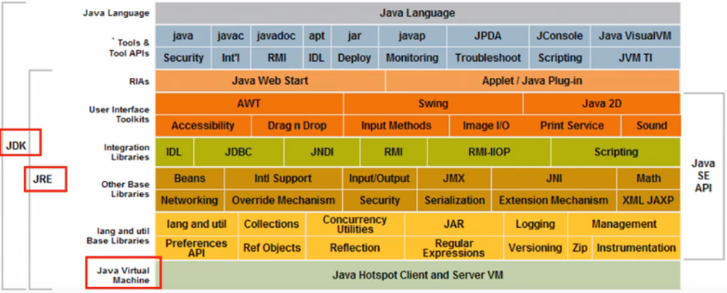# Java开发环境搭建

• JDK下载与安装
• 配置环境变量
• JDK目录介绍
• HelloWorld及简单语法规则

### 卸载JDK

• 删除Java的安装目录
• 删除JAVA_HOME
• 删除path下关于Java的目录
• cmd输入java -version（有空格）

### 配置环境变量

JAVA_HOMEJava安装的路径文件夹
PathJAVA_HOMEbinJAVA_HOME\jre\bin

# HelloWorld

1. 新建一个文件夹，存放代码【该目录下的code目录】
2. 新建Java文件
• 文件后缀名为java
• Hello.java
• 【注意点】系统可能没有显示文件后缀名
1. 编写代码
``````public class Hello {
public static void main(String[] args) {
System.out.println("Hello World!");
}
}``````
1. 编译javac java文件
2. 运行class文件，java class文件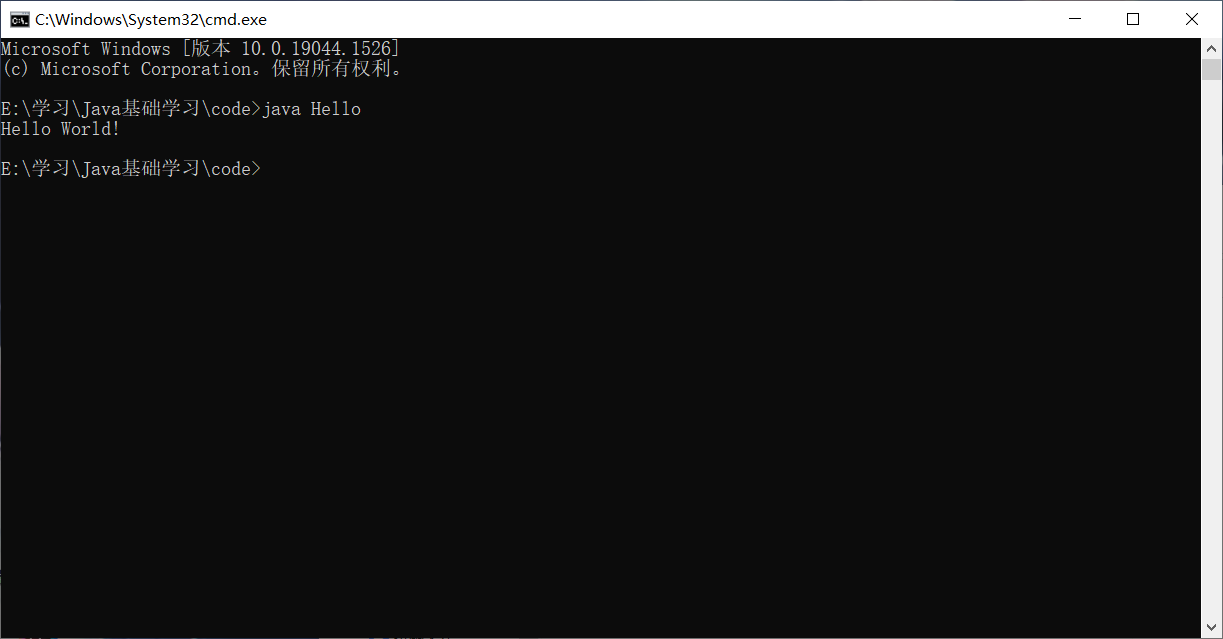### 可能会遇到的情况

1. 每个单词的大小不能出现问题，Java是大小写敏感的
2. 尽量使用英文
3. 文件名和类名必须保证-致,I 并且首字母大写

# 编译型和解释型

• 编译型
• 解释型

• 程序运行机制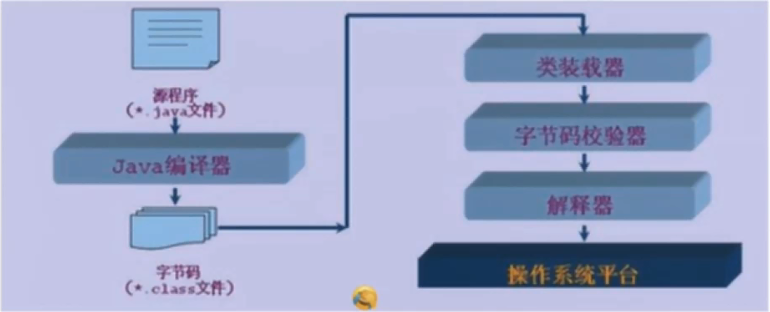# 注释

• 平时我们编写代码，在代码量比较少的时候，我们还可以看懂自己写的,但是当项目结构一旦复杂起来，我们就需要用到注释了。
• 注释并不会被执行，是给我们写代码的人看的
• 书写注释是一个非常好的习惯

• Java中的注释有3种:

• 单行注释
• 多行注释
• 文档注释
``````public class HelloWorld {
public static void main(String[] args) {
//输出一个HelloWorld
// 单行注释：只能注释一行文字
System.out.println("HelloWorld");
/*
* 输出一个HelloWorld
* 多行注释：注释段落文字
*/
System.out.println("HelloWorld");
/**
* @Description HelloWorld
*这是文档注释
* */
}
}``````

# 数据类型

• 强类型语言

• 要求变量的使用要严格符合规定，所有变量都必须先定义后才能使用
• 弱类型语言
• Java的数据类型分为两大类

• 基本类型(primitive type)
• 引用类型(reference type)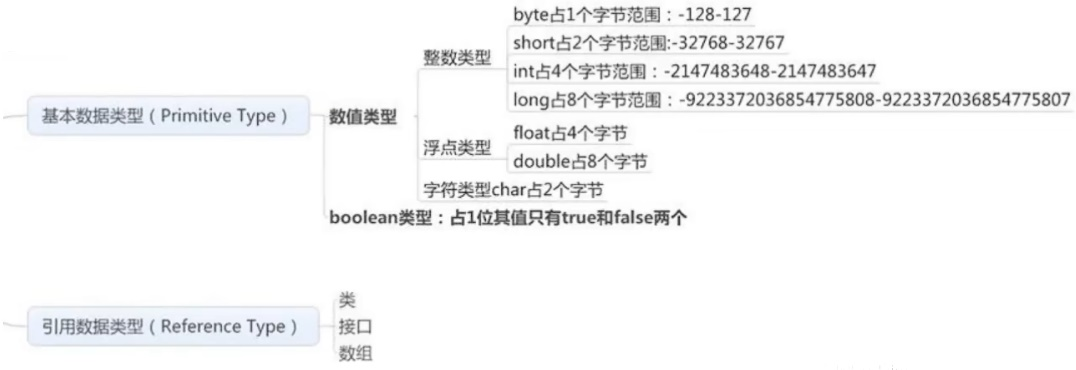## 变量

• 变量是什么:就是可以变化的量!
• Java是一种强类型语言，每个变量都必须声明其类型。
• Java变量是程序中最基本的存储单元，其要素包括变量名,变量类型和作用域。

``````type varName[=value] [{, varName[=value]}] ;
//数据类型 变量名 = 值; 可以使用逗号隔开来声明多个同类型变量。
``````
• 注意事项:

• 每个变量都有类型，类型可以是基本类型，也可以是引用类型。
• 变量名必须是合法的标识符。
• 变量声明是-条完整的语句，因此每一-个声明都必须以分号结束

## 什么是字节

• 位(bit) :是计算机内部数据储存的最小单位, 11001100是一个八位二进制数。
• 字节(byte) :是计算机中数据处理的基本单位，习惯.上用大写B来表示，
• 1B (byte,字节) = 8bit (位)
• 字符:是指计算机中使用的字母、数字、字和符号
• 1 bit示1位
• 1Byte表示一个字节1B=8b
• 1024B=1KB
• 1024KB= 1 M
• 1024M=1G

1. 计算能力不同：64位的系统理论上比32位系统快一倍，并且它们的内存寻址也不一样。
2. 支持的最大运行内存不同：32位的电脑最大只支持4G（一般情况只能用3.25G左右），而64位的电脑则可以支持128G甚至更大
3. 运行的软件不同：32位的电脑只能运行32位的软件，而64位的电脑可以运行32位的软件也可以运行64位的软件。
4. 支持的系统不同：32位电脑支持32位的系统，而64位的电脑支持支持32位的系统也支持64位的系统。

# 数据类型扩展及面试题讲解

``````public class Demo03 {
public static void main(String[] args) {
//整数拓展: 进制 二进制0b 十进制 八进制0 六进制0x
int i=10;
int i2 = 010; //八进制0
int i3 = 0x10; //十六进制0x 0~9 A~F 16

System.out.println(i);
System.out.println(i2);
System.out.println(i3);
System.out.println("===============================");
//================================================================
//浮点数拓展?  银行业务怎么表示?钱
//BigDecimal数学 工具类
//================================================================
//float 有限 离散 舍入误差 大约 接近但不等于
//doubLe
//最好完全使用浮点数进行比较

float f = 0.1f; //0.1
double d = 1.0/10; //0.1

System.out.println(f==d); //false

float d1 = 23131312312312313f;
float d2=d1+1;
System.out.println(d1==d2); //true

//===================================================================
//字符拓展?
//===================================================================
System.out.println("===============================");

char c1 = 'a';
char c2 = '中';

System.out.println(c1);
System.out.println((int)c1); //强制换行

System.out.println(c2);
System.out.println((int)c2); //强制换行
//所有的字符本质还是数字
//编码 Unicode表:(97=a 65=a) 2字节 0-65536 ExceL 2 16=65536

char c3 ='\u0061' ;
System. out. println(c3); //a

//转义字符
// \t 制表符
// \n 换行
//.....
System . out. println( "He1l1o\nWor1d");

System.out.println("=================================");
String sa = new String("hel1o world");
String sb = new String("hello world");
System.out.println(sa==sb);
String sc = "he1lo world";
String sd = "he11o world";
System.out.println(sc==sd);
//对象从内存分析

//布尔值扩展
boolean flag = true;
if (flag==true){} //新手
if (flag){} //老手
//Less is More!代码要精简易读

}
}
``````

# 类型转换

• 由于Java是强类型语言，所以要进行有些运算的时候的，需要用到类型转换。
``````低----------------------------->高
byte,short,char->int->1ong->float->double``````
• 运算中，不同类型的数据先转化为同一类型，然后进行运算。

• 强制类型转换
• 自动类型转换

# 变量，常量，作用域

### 变量

• 变量是什么:就是可以变化的量!
• Java是-种强类型语言,每个变量都必须声明其类型。
• Java变量是程序中最基本的存储单元，其要素包括变量名，变量类型和作用域

``````type varName [ =value] [{,varName[=value]}] ;
//数据类型变量名 =值;可以使用逗号隔开来声明多个同类型变量。``````
• 注意事项:

• 每个变量都有类型，类型可以是基本类型，也可以是引用类型。
• 变量名必须是合法的标识符。
• 变量声明是一条完整的语句，因此每一个声明都必须以分号结束

### 变量作用域

• 类变量
• 实例变量
• 局部变量

``````public class Variable{
static int allClicks=0;//类变量
String str="hello world"; // 实例变量

public void method(){
int i =0; //局部变量
}
}``````

### 常量

• 常量(Constant):初始化(initialize)后不能再改变值!不会变动的值。
• 所谓常量可以理解成-种特殊的变量，它的值被设定后，在程序运行过程中不允许被改变。

``````final常量名=值;
final double PI=3.14;``````
• 常量名- -般使用大写字符。

### 变量的命名规范

• 所有变量、方法、类名:见名知意
• 类成员变量:I首字母小写和驼峰原则: monthSalary （除了第一个单词以外，后面单词首字母大写）
• 局部变量:首字母小写和驼峰原则
• 常量:大写字母和下划线: MAX_VALUE
• 类名:首字母大写和驼峰原则: Man, GoodMan
• 方法名: 首字母小写和驼峰原则: run(), runRun()

### 变量作用域

• 类变量
• 实例变量
• 局部变量

``````public class Variable{
static int allClicks=0;//类变量
String str="hello world"; // 实例变量

public void method(){
int i =0; //局部变量
}
}``````

# 运算符

### Java语言支持如下运算符:

• 算术运算符:+, -.*，/, %，++, -
• 赋值运算符=
• 关系运算符:>, <，>=, <=, ==，!= instanceof
• 逻辑运算符:&&, ||， !
• 位运算符:&, |, ^, ~，》>，<<, >>>(了解! ! ! )
• 条件运算符? :
• 扩展赋值运算符:+=，-=, *=, /=

### 运算符优先级

1括号()、[ ]、.
2非、正，负号!、+(正)、-(负)
3自增，自减++、--
4乘，除，取余*、/、%
5加，减+、-
6移位运算<<、>>、>>>
7大小关系<、<=、>、>=
8相等关系==、!=
9按位与&
10按位异或（在java中，这不是幂次方的符号）^
11按位或\
12逻辑与(短路与)&&
13逻辑或(短路或)\\
14条件运算(三目运算)? :
15赋值运算=、+=、-=、*=、/=、%=
16位赋值运算&=、\=、^=、~=、<<=、>>=、>>>=

### 基本运算符

``````public class Demo01 {
public static void main(String[] args) {
//二元运算符
//Ctrl+D :复制当前行到下一行
int a=10;
int b=20;
int C=25;
int d=25;
System.out.println(a+b);
System.out.println(a-b);
System.out.println(a*b);
System.out.println(a/(double)b);

}
}``````
``````public class Demo02 {
public static void main(String[] args) {
long a = 123123123123123L;
int b = 123;
short c = 10;
byte d=8;
System.out.println(a+b+c+d); //Long
System.out.println(b+c+d); //Int
System.out.println(c+d); //Int

}
}``````
``````package operator;

public class Demo03 {
public static void main(String[] args) {
//关系运算符返回的结果:正确， 错误布尔值
//if

int a=10;
int b=20;
int c=21;

//取余数，模运算
System.out.println(c%a); //c / a 21/10=2...1

System . out. println(a>b);
System. out .println(a<b);
System . out. println(a==b);

}
}``````

### 自增自减运算符，初识Math类

``````public class Demo04 {
public static void main(String[] args) {
//++ --  自增，自减  一元运算符
int  a=3;
int b = a++; //执行完这行代码后,先给b赋值,再自增
//a++ a=a+1
int c= ++a; //执行完这行代码后,先自增,再给c赋值
//++a a=a+1
System. out . println(a);
System. out. println(b);
System. out . println(c);

//幂运算2^3 22*2=8 很多运算，我们会使用一-些工具类来操作!
double pow = Math. pow(3, 2);
System.out.println(pow);

}
}``````

### 逻辑运算符、位运算符

``````//逻辑运算符
public class Demo05 {
public static void main(String[] args) {
//与(and)或(or)非(取反)
boolean a = true;
boolean b = false;
System.out.println("a && b: "+(b&&a)); //逻辑与运算:两个变量都为真，结果才为true
System.out.println("a | b:"+(a||b)); //逻辑或运算: 两个变量有一一个为真，则结果才为true
System.out.println("! (a && b) :"+!(a&&b)); //如果是真，则变为假，如果是假则变为真
//短路运算
int c=5;
boolean d = (c<4)&&(c++<4);
System.out.println(d);
System.out.println(c);

}
}``````
``````//位运算符
public class Demo06 {
public static void main(String[] args) {
/*
A = 0011 1100
B = 0000 1101
------------------------
A&B = 0000 1100
A/B = 0011 1101
A^B = 0011 0001
~B = 1111 0010
2*8 = 16 2*2*2*2
效率极高！！！
<< *2
>> /2
0000 0000 0
0000 0001 1
0000 0010 2
*/
System.out.println(2<<3);
}
}
``````

### 三元运算符

``````//三元运算符
public class Demo08 {
public static void main(String[] args) {
//x ? y : z
//如果x==true，则结果为y，否则结果为z

int score = 50;
String type =score <60 ?"不及格":"及格";
//if
System. out . println(type);

}
}``````

# 包机制

• 为了更好地组织类, Java 提供了包机制，用于区别类名的命名空间。
• 包语句的语法格式为:
``package pkg1[. pkg2[. plkg...]];``
• 一般利用公司域名倒置作为包名;
• 为了能够使用某-个包的成员,我们需要在Java程序中明确导入该包。使用"import"语句可
完成此功能
``import package1[ . package2..] . (classname| *);``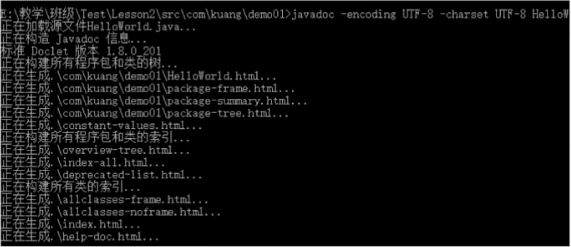• 参数信息

• @author作者名
• @version版本号
• @since 指明需要最早使用的jdk版本
• @param 参数名
• @return返回值情况
• @throws 异常抛出情况
``javadoc -encoding UTF-8 -charset UTF-8 【java文件】``

``````/**
* @author Xiang
* @version 1.0
* @since 1.8
* */
public class Doc {
String name;

/**
* @author Xiang
* @param name
* @return
* @throws Exception
*/
public String test(String name) throws Exception{
return name;
}
}``````

# 用户交互 Scanner

### Scanner对象

• 之前我们学的基本语法中我们并没有实现程序和人的交互，但是Java给我们提供了这样一 个工具类,我们可以获取用户的输入。java.util.Scanner 是Java5的新特征，我们可以通过Scanner类来获取用户的输入。
• 基本语法:
``Scanner S = new Scanner(System. in);``
• 通过Scanner类的next()与nextLine()方法获取输入的字符串,在读取前我们一般需要使用hasNext()与hasNextLine()判断是否还有输入的数据。

### 示例代码：

``````import java.util.Scanner;

public class Demo01 {
public static void main(String[] args) {
//创建- -个扫描器对象，用于接收键盘数据
Scanner scanner = new Scanner(System. in);
System.out.println(" 使用next方式接收: ");
//判断用户有没有输入字符串
if (scanner .hasNext()){
//使用next方式接收
String str = scanner .next();
System.out.println("输出的内容为: "+str);
}
//凡是属于I0流的类如果不关闭会一直 占用资源.要养成好习惯用完就关掉
scanner.close();
}
}``````
``````import java.util.Scanner;

public class Demo01 {
public static void main(String[] args) {
//从键盘接收数据
Scanner scanner = new Scanner(System.in);
System.out.println("请输入数据: ");
String str = scanner.nextLine();
System.out.println("输出的内容为: "+str);
scanner.close();
}
}``````

# Scanner进阶使用

### 判断是否为整数：

``````import java.util.Scanner;

public class Demo03 {
public static void main(String[] args) {
Scanner scanner = new Scanner(System. in);
//从键盘接收数据!
int i=0;
float f = 0.0f;
System.out.println("请输入整数: ");
//如果...那么
if (scanner.hasNextInt()){
i = scanner.nextInt( );
System.out.println("整数数据: " + i);
}else {
System.out .println("输入的不是整数数据! ");
}
System.out.println("请输入小数: ");
//如果...那么
if (scanner.hasNextFloat()){
f = scanner. nextFloat();
System.out.println("小数数据: " + f);
}else {
System.out.println("输入的不是小数数据! ");
}

}
}``````

### 计算器：判断是否为数字，如果不是则算出最终结果

``````import java.util.Scanner;

public class Demo04 {
public static void main(String[] args) {
//我们可以输入多个数字，并求其总和与平均数，每输入一个数字用回车确认，通过输入非数字来结束输入并输出执行结果:
Scanner scanner = new Scanner(System.in);
//和
double sum = 0;
//计算输入了多少个数字
int m=0;
System.out.println("请输入数据：");
//通过循环判断是否还有输入，并在里面对每一次进行求和和统计
while (scanner.hasNextDouble()) {
double X = scanner.nextDouble();
m = m + 1;//m++
sum = sum + X;
System.out.println("你输入了第"+m+"个数据，然后当前结果sum="+sum);
}
System.out.println(m + "个数的和为" + sum);
System.out.println(m + "个数的平均值是" + (sum / m));

scanner.close();
}
}
``````

# 顺序结构

• JAVA的基本结构就是顺序结构，除非特别指明，否则就按照顺序一句一句执行。
• 顺序结构是最简单的算法结构。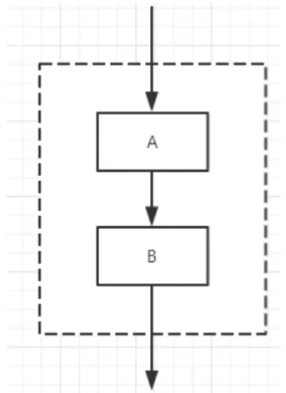• 语句与语句之间，框与框之间是按从上到下的顺序进行的，它是由若干个依次执行的处理步骤组成的，它是任何一个算法都离不开的一种基本算法结构。

### 示例代码：

``````  public class ShunXuDome {

public static void main(String[] args) {
//代码是由上到下运行
System.out.println("hello1");
System.out.println("hello2");
System.out.println("hello3");
System.out.println("hello4");
System.out.println("hello5");
}
}``````

# 选择结构

• if单选择结构
• if双选择结构
• if多选择结构
• 嵌套的if结构
• switch多选择结构

### if单选择结构

• 我们很多时候需要去判断-个东西是否可行,然后我们才去执行，这样-个过程在程序中用if语句来表示
• 语法
``````if(布尔表达式){
//如果布尔表达式为true将执行的语句
}``````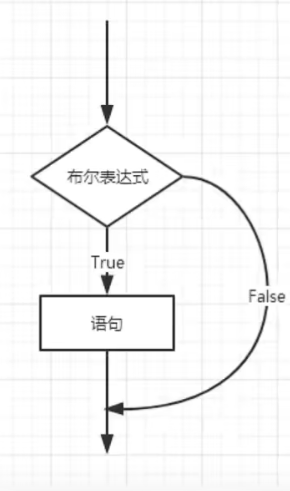#### 示例代码：

``````import java.util.Scanner;

public class IfDemo {
public static void main(String[] args) {
Scanner scanner = new Scanner(System. in);
System.out.println("请输入内容: ");
String s = scanner.nextLine( );
//equals:判断字符串是否相等
if (s.equals("hel1o")){
System.out.println(s);
}
System.out.println("End");
scanner.close();
}
}``````

### if双选择结构

• 那现在有个需求，公司要收购- -个软件，成功了,给人支付100万元，失败了，自己找人开发。
• 这样的需求用一个if就搞不定了，我们需要有两个判断，需要一个双选择结构，所以就有了if-else结构。
• 语法

``````if(布尔表达式){
//如果布尔表达式的值为true
}else{
//如果布尔表达式的值为false
}``````

![if双选择结构](img/if双选择结构.png)

#### 示例代码

``````  import java.util.Scanner;

public class IfDemo02 {
public static void main(String[] args) {
//考试分数大于6θ就是及格，小于60分就不及格。
Scanner scanner = new Scanner(System.in);
System.out.println("请输入成绩: ");
int score = scanner.nextInt();
if (score > 60) {
System.out.println("及格");
} else {
System.out.println("不及格");
scanner.close();
}
}
}``````

### if多选择结构

• 我们发现刚才的代码不符合实际情况，真实的情况还可能存在ABCD,存在区间多级判断。比如
90-100就是A, 80-90就是B..等等,在生活中我们很多时候的选择也不仅仅只有两个，所以我
们需要一-个多选择结构来处理这类问题!
• 语法：

``````if(布尔表达式1){
![if多选择结构](img/if多选择结构.png)//如果布尔表达式1的值为true执行代码
}else if(布尔表达式2){
//如果 布尔表达式2的值为true执行代码
}else if(布尔表达式3){
//如果布尔表达式 3的值为true执行代码
}else {
//如果以 上布尔表达式都不为true执行代码
}``````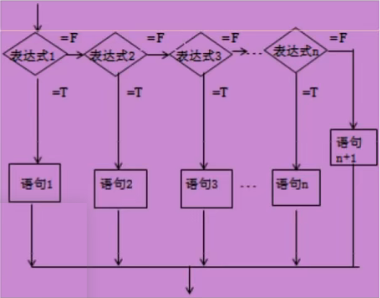#### 示例代码

``````import java.util.Scanner;

public class IfDemo03 {
public static void main(String[] args) {
//考试分数大于60就是及格，小于60分就不及格。
Scanner scanner = new Scanner(System.in);
System.out.println("请输入成绩: ");
int score = scanner.nextInt();
if (score == 100) {
System.out.println("恭喜满分");
} else if (score < 100 & score >= 90) {
System.out.println("A级");
} else if (score < 90 && score >= 80) {
System.out.println("B级");
} else if (score < 80 && score >= 70) {
System.out.println("C级");
} else if (score < 70 && score >= 60) {
System.out.println("D级");
} else if (score <= 60 && score >= 0) {
System.out.println("不及格");
} else {
System.out.println("成绩不合法");
}
scanner.close();
}
}``````

### 嵌套的if结构

• 使用嵌套的if..else语句是合法的。也就是说你可以在另-个if或者else if语句中使用if或者else if语句。你可以像if语句-样嵌套else if..else。
• 语法:
``````if(布尔表达式1){
////如果布尔表达式1的值为true执行代码
if(布尔表达式2){
////如果布尔表达式2的值为true执行代码
}
}``````

### 思考?我们需要寻找一个数，在1-100之间?

``````public class IfDemo04 {
public static void main(String[] args) {
//获取一个随机数
//double d = Math.random();
//System.out.println(d);

//需求：我要获取一个1-100之间的随机数，肿么办?
for (int x = 0; x < 100; x++) {
int number = (int) (Math.random() * 100) + 1;
System.out.println(number);
}
}
}``````

### Switch选择结构

• 多选择结构还有一个实现方式就是switch case语句。
• switch case语句判断一个变量与一系列值中某个值是否相等，每个值称为一个分支。

• switch语句中的变量类型可以是:

• byte、short, int 或者char.
• 从JavaSE7开始
• switch支持字符串String类型了
• 同时case标签必须为字符串常量或字面量。
• 语法：

``````switch(expression){
case value :
//语句
break; //可选
case value :
//语句
break; //可选
//你可以有任意数量的case语句
default : //可选
//语句
}``````

#### 示例代码

``````public class SwitchDemo01 {
public static void main(String[] args) {
//case穿透//switch 匹配一个具体的值
case 'A':
System.out.println("优秀");
break; // 可选
case 'B':
System.out.println("良好");
case 'C':
System.out.println("及格");
case 'D':
System.out.println("再接再厉");
case 'E':
System.out.println("挂科");
default:
System.out.println("未知等级");
}
}
}

​# 循环结构

• while循环
• do...while循环
• for循环
• 在Java5中引入了一种主要用于数组的增强型for循环。

### while循环

• while是最基本的循环，它的结构为:
``````while(布尔表达式){
//循环内容
}``````
• 只要布尔表达式为true，循环就会一直执行下去。
• 我们大多数情况是会让循环停止下来的，我们需要一个让表达式失效的方式来结束循环。少部分情况需要循环一直执行，比如服务器的请求响应监听等。
• 循环条件一直为true就会造成无限循环【死循环】，我们正常的业务编程中应该尽量避免死循环。会影响程序性能或者造成程序卡死奔溃!
• 思考:计算1+2+3+...+100=?
``````public class WhileDemo03 {
public static void main(String[] args) {
//计算1+2+3+...+100=?

//高斯的故事

int i = 0;
int sum = 0;
while (i<=100){
sum =sum + i;
i++;
}
System.out.println(sum) ;

}
}``````

# do...while循环

### do...while循环

• 对于while语句而言，如果不满足条件，则不能进入循环。但有时候我们需要即使不满足条件，也至少执行一次。
• do...while循环和while循环相似，不同的是，do...while循环至少会执行一次。
``````do {
//代码语句
}while(布尔表达式);``````
• While和do-While的区别:

• while先判断后执行。dowhile是先执行后判断!
• Do...while总是保证循环体会被至少执行一次!这是他们的主要差别。
``````public class DoWhileDemo02 {
public static void main(String[] args) {
int a = 0;
while (a<0){
System.out.println(a);
a++;
}
System.out.println("==============");
do {
System.out.println(a);
a++;
}while (a<0);

}
}
//输出：============== \n 0``````

# For循环

• 虽然所有循环结构都可以用while或者do...while表示，但Java提供了另一种语句——for循环，使一些循环结构变得更加简单。
• for循环语句是支持迭代的一种通用结构，是最有效、最灵活的循环结构。
• for循环执行的次数是在执行前就确定的。语法格式如下:
``````for(初始化;布尔表达式;更新){
//代码语句
}``````

### 练习1∶计算0到100之间的奇数和偶数的和

``````  public class ForDemo02 {
public static void main(String[] args) {
//计算0到100之间的奇数和偶数的和
int oddSum = 0;
int evenSum = 0;
for (int i = 0; i <= 100; i++) {
if (i%2!= 0) {
oddSum += i;
} else {
evenSum += i;
}
}
System.out.println("奇数的和:"+oddSum);
System.out.println("偶数的和:" + evenSum);
}
}``````

### 练习2:用while或for循环输出1-1000之间能被5整除的数，并且每行输出3个

``````public class ForDemo03 {
public static void main(String[] args) {
//用while或for循环输出1-1000之间能被5整除的数，并且每行输出3个
for (int i = 0; i <= 1000; i++) {
if (i%5==0){
System.out.print(i+"\t");
}
if (i%(5*3)==0){ //每行
System.out.println();
}
}
}
}``````

### 练习3:打印九九乘法表

``````public class ForDemo04 {
public static void main(String[] args) {
// 打印九九乘法表
for (int j = 1; j <= 9; j++) {
for (int i = 1; i <=j; i++) {
System.out.print(j+"*"+i+"="+(j*i)+'\t');
}
System.out.println();
}
}
}``````

## 增强for循环

• 这里我们先只是见一面，做个了解，之后数组我们重点使用
• Java5引入了一种主要用于数组或集合的增强型for循环。
• Java增强for循环语法格式如下:
``````for(声明语句︰表达式){
//代码句子
}``````
• 声明语句:声明新的局部变量，该变量的类型必须和数组元素的类型匹配。其作用域限定在循环语句块，其值与此时数组元素的值相等。
• 表达式:表达式是要访问的数组名，或者是返回值为数组的方法。
``````public class ForDemo05 {
public static void main(String[] args) {
int[] numbers = {10, 20, 30, 40, 50}; // 定义了一个数组
for (int i = 0; i < numbers.length; i++) { //numbers.length 数组的长度
//读取数组
System.out.println(numbers[i]);
}
}
}``````

# break continue

• break在任何循环语句的主体部分，均可用break控制循环的流程。break用于强行退出循环，不执行循环中剩余的语句。(break语句也在switch语句中使用)
• continue语句用在循环语句体中，用于终止某次循环过程，即跳过循环体中尚未执行的语句，接着进行下一次是否执行循环的判定。

• 关于goto关键字

• goto关键字很早就在程序设计语言中出现。尽管goto仍是Java的一个保留字，但并未在语言中得到正式使用;Java没有goto。然而，在break和continue这两个关键字的身上，我们仍然能看出一些goto的影子---带标签的break和continue
• “标签”是指后面跟一个冒号的标识符，例如: label:
• 对Java来说唯一用到标签的地方是在循环语句之前。而在循环之前设置标签的唯一理由是:我们希望在其中嵌套另一个循环，由于break和continue关键字通常只中断当前循环，但若随同标签使用，它们就会中断到存在标签的地方。

# 打印三角形及Debug

### 代码如下：

``````public class TestDemo {
public static void main(String[] args) {
//打印三角形5行

for (int i = 1; i <= 5; i++) {
for ( int j = 5; j >=i ; j--) {
System.out.print(" ");
}
for (int j = 1; j <=i ; j++) {
System.out.print("*");
}
for (int j = 1; j < i; j++) {
System.out.print("*");
}
System.out.println();
}
}
}``````

### debug的意思：

1. 排除故障，排除错误；
2. 调整程序，移去程序中的错误；
3. 除害虫，除去建筑物内的窃听器。
4. 程序故障统称为“臭虫（BUG）”，把排除程序故障叫DEBUG，而这奇怪的“称呼”，成为后来计算机领域的专业行话。

# 什么是方法

### 何谓方法？

• System.out.println()，那么它是什么呢?

• Java方法是语句的集合，它们在一起执行一个功能。
-方法是解决一类问题的步骤的有序组合

• 方法包含于类或对象中
• 方法在程序中被创建，在其他地方被引用
• 设计方法的原则:方法的本意是功能块，就是实现某个功能的语句块的集合。我们设计方法的时，最好保持方法的原子性，就是一个方法只完成1个功能，这样利于我们后期的扩展
• 回顾:方法的命名规则?

• 方法的名字的第一个单词应以小写字母作为开头，后面的单词则用大写字母开头。
• 示例：demoFunction

### 方法示例：

``````public class Demo01 {
//main 方法
public static void main(String[] args) {
}
//加法
public static int add(int a,int b){
return a+b;
}
}``````

# 方法的定义和调用

### 方法的定义

• Java的方法类似于其它语言的函数，是一段用来完成特定功能的代码片段，一般情况下，定义一个方法包含以下语法:
• 方法包含一个方法头和一个方法体。下面是一个方法的所有部分:

• 修饰符：修饰符，这是可选的，告诉编译器如何调用该方法。定义了该方法的访问类型。
• 返回值类型∶方法可能会返回值。returnValueType 是方法返回值的数据类型。有些方法执行所需的操作，但没有返回值。在这种情况下，returnValueType是关键字void。
• 方法名:是方法的实际名称。方法名和参数表共同构成方法签名。
• 参数类型:参数像是一个占位符。当方法被调用时，传递值给参数。这个值被称为实参或变量。参数列表是指方法的参数类型、顺序和参数的个数。参数是可选的，方法可以不包含任何参数。

• 形式参数:在方法被调用时用于接收外界输入的数据。
• 实参:调用方法时实际传给方法的数据。
• 方法体:方法体包含具体的语句，定义该方法的功能。
``````修饰符 返回值类型 方法名(参数类型 参数名){
...
方法体
...
return 返回值;
}``````

### 方法调用

• 调用方法:：对象名.方法名(实参列表)
• Java支持两种调用方法的方式，根据方法是否返回值来选择。当方法返回一个值的时候，方法调用通常被当做一个值。例如:
``int larger = max( 30，40);``
• 如果方法返回值是void，方法调用一定是一条语句。
``system.out.println( "Hello,kuangshen! ");``

# 方法的重载

• 重载就是在一个类中，有相同的函数名称，但形参不同的函数。
• 方法的重载的规则:

• 方法名称必须相同。
• 参数列表必须不同（个数不同、或类型不同、参数排列顺序不同等)。
• 方法的返回类型可以相同也可以不相同
• 仅仅返回类型不同不足以成为方法的重载。
• 实现理论:

• 方法名称相同时，编译器会根据调用方法的参数个数、参数类型等去逐个匹配，以选择对应的方法，如果匹配失败，则编译器报错。

# 命令行传参

• 有时候你希望运行一个程序时候再传递给它消息。这要靠传递命令行参数给main()函数实现。

``````public class CommandLine {
public static void main(String args[]){
for(int i=o; i<args.length; i++){
system.out.println( "args[" + i + "]:" + args[i]);
}
}
}``````

# 可变参数

• JDK 1.5开始，Java支持传递同类型的可变参数给一个方法。
• 在方法声明中，在指定参数类型后加一个省略号(.…)。
• 一个方法中只能指定一个可变参数，它必须是方法的最后一个参数。任何普通的参数必须在它之前声明。
``````public static void printMax( double. . . numbers) {
if (numbers.length == 0) {
System.out.println("No argument passed" );
return;
}
double result = numbers;
//排序!
for (int i = 1; i <numbers.length; i++){
if (numbers[i] >result) {
result = numbers[i];
}
}
System.out.println( "当前数组最大的值是：" + result);
}``````

# 递归

• A方法调用B方法，我们很容易理解!
• 递归就是:A方法调用A方法!就是自己调用自己
• 利用递归可以用简单的程序来解决一些复杂的问题。它通常把一个大型复杂的问题层层转化为一个与原问题相似的规模较小的问题来求解，递归策略只需少量的程序就可描述出解题过程所需要的多次重复计算，大大地减少了程序的代码量。递归的能力在于用有限的语句来定义对象的无限集合。
• 递归结构包括两个部分:

• 递归头:什么时候不调用自身方法。如果没有头，将陷入死循环。
• 递归体:什么时候需要调用自身方法。

### 示例代码：

``````public class Demo06 {
public static void main(String[] args) {
System.out.println(3); //输出 3
}
//1! 1
//2! 2*1
public static int f(int n){
if (n==1){
return 1;
}else {
return n*f(n-1);
}
}
}``````

# 什么是数组

### 数组的定义

• 数组是相同类型数据的有序集合.
• 数组描述的是相同类型的若干个数据,按照一定的先后次序排列组合而成。
• 其中,每一个数据称作一个数组元素,每个数组元素可以通过一个下标来访问它们.

### 数组声明创建

• 首先必须声明数组变量，才能在程序中使用数组。下面是声明数组变量的语法:
``````dataType[ ] arrayRefVar; //首选的方法

dataType arrayRefVar[]; //效果相同，但不是首选方法``````
• Java语言使用new操作符来创建数组，语法如下:
``dataType[] arrayRefVar = new dataType[arraySize];``
• 数组的元素是通过索引访问的，数组索引从О开始。
获取数组长度:
``arrays.length``

#### 示例代码：

``````public class ArrayDemo01 {
// 变量的类型 变量的名字=变量的值;
// 数组类型
public static void main(String[] args) {
// 1.两种定义方式
int[] nums1;
int nums2[];

nums1=new int; //2.这里面可以存放10个int类型的数字

//3.给数组元素中赋值
nums1=1;
nums1=2;
nums1=3;
nums1=4;
nums1=5;

System.out.println(nums1); //输出 1

//计算所有元素的和
int sum=0;
//获取数组长度：nums1.length
for (int i = 0; i < nums1.length; i++) {
sum=sum+nums1[i];
}
System.out.println(sum);
}
}``````

### 数组的四个基本特点

• 其长度是确定的。数组一旦被创建，它的大小就是不可以改变的。
• 其元素必须是相同类型,不允许出现混合类型。
• 数组中的元素可以是任何数据类型，包括基本类型和引用类型。
• 数组变量属引用类型，数组也可以看成是对象，数组中的每个元素相当于该对象的成员变量。数组本身就是对象，Java中对象是在堆中的，因此数组无论保存原始类型还是其他对象类型,数组对象本身是在堆中的。

### 数组边界

• 下标的合法区间[O, length-1]，如果越界就会报错;
``````public static void main(String[ ] args) {
int[] a=new int;
system.out.println(a);
}``````
• ArraylndexOutOfBoundsException :数组下标越界异常!
• 小结:

• 数组是相同数据类型(数据类型可以为任意类型)的有序集合数组也是对象。
• 数组元素相当于对象的成员变量
• 数组长度的确定的，不可变的。如果越界，则报:ArraylndexOutofBounds

# 数组使用

• 普通的for循环
• For-Each循环
• 数组作方法入参
• 数组作返回值
``````public class ArrayDemo04 {
public static void main(String[] args) {
int[] arrays={1,2,3,4,5};

//JDK1.5, 没有下标
for(int array:arrays){
System.out.println(array);
}

System.out.println("============");
printArray(arrays);
System.out.println("============");
int[] reverse= reverse(arrays);
printArray(reverse);
}
//打印数组元素
public static void printArray(int[] arrays){
for (int i = 0; i < arrays.length; i++) {
System.out.println(arrays[i]);
}
}

//反转数组元素
public static int[] reverse(int[] arrays){
int[] result=new int[arrays.length];

//反转操作
for (int i=0,j= result.length-1;i<arrays.length;i++,j--){
result[j]=arrays[i];
}
return result;
}
}``````

# 二位数组

### 多维数组

• 多维数组可以看成是数组的数组，比如二维数组就是一个特殊的一维数组，其每一个元素都是一个一维数组。
• 二维数组
``int a[][] = new int;``
• 解析:以上二维数组a可以看成一个两行五列的数组。
• 思考:多维数组的使用?
``````public class ArrayDemo05 {
public static void main(String[] args) {
int[][] array={{1,2,3},{2,3},{3,4},{4,5}};
System.out.println(array); //输出{1,2,3}中的3

System.out.println("===============");

//进阶for读取数组元素
for (int i = 0; i < array.length; i++) {
for (int j = 0; j < array[i].length; j++) {
System.out.println(array[i][j]);
}
}
}
}``````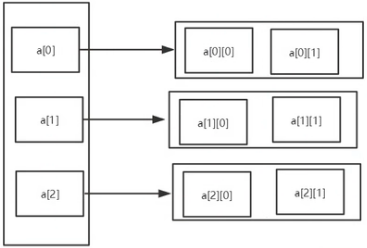# Arrays类

• 数组的工具类java.util.Arrays
• 由于数组对象本身并没有什么方法可以供我们调用,但API中提供了一个工具类Arrays供我们使用,从而可以对数据对象进行一些基本的操作。
• 查看JDK帮助文档
• Arrays类中的方法都是static修饰的静态方法,在使用的时候可以直接使用类名进行调用,而"不用"使用对象来调用(注意:是"不用"而不是"不能")

• 具有以下常用功能:

• 给数组赋值:通过fill方法。
• 对数组排序:通过sort方法,按升序。
• 比较数组:通过equals 方法比较数组中元素值是否相等。
• 查找数组元素:通过binarySearch方法能对排序好的数组进行二分查找法操作。

### 示例代码：

``````import java.util.Arrays;

public class ArrayDemo06 {
public static void main(String[] args) {
int[] a = {1,2,3,4,9090,31231,543,21,3,23};
System.out.println(a); // [I@4554617c
//打印数组元素Arrays.tostring
System.out.println(Arrays.toString(a)); //输出 [1, 2, 3, 4, 9090, 31231, 543, 21, 3, 23]

Arrays.sort(a); // 数组进行排序 升序
System.out.println(Arrays.toString(a));
}
}``````

# 冒泡排序

• 冒泡排序无疑是最为出名的排序算法之一，总共有八大排序!
• 冒泡的代码还是相当简单的，两层循环，外层冒泡轮数，里层依次比较，江湖中人人尽皆知
• 我们看到嵌套循环，应该立马就可以得出这个算法的时间复杂度为O(n2)。
思考:如何优化?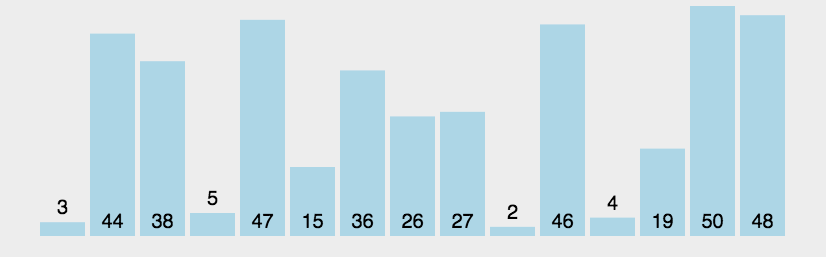### 示例代码：

``````import java.util.Arrays;

public class ArrayDemo07 {
public static void main(String[] args) {
int[] a = {1, 4, 54, 4, 12, 7};

int[] sort = sort(a);
System.out.println(Arrays.toString(sort));
}

//冒泡排序
//1.比较数组中，两个相邻的元素，如果第一个比第二个数大，我们就交换他们的位置
//2.每一次比较，都会产生出一个最大，或者最小的数字;
//3.下一轮则可以少一次排序!
//4.依次新环，直到结柬!
public static int[] sort(int[] array) {
//临时变量
int temp = 0;

//外层循环，判断我们这个要走多少次;
for (int i = 0; i < array.length - 1; i++) {
//内层循环，比价判断两个数，如果第一个数，比第二个数大，则交换位置
for (int j = 0; j < array.length - 1 - i; j++) {
//将数组元素从大到小排序 如果为 < 结果则相反
if (array[j + 1] > array[j]) {
temp = array[j];
array[j] = array[j + 1];
array[j + 1] = temp;
}
}
}
return array;
}
}``````

# 稀疏数组

• 需求:编写五子棋游戏中，有存盘退出和续上盘的功能。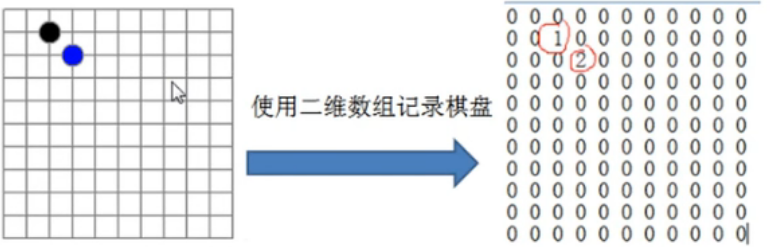• 分析问题:因为该二维数组的很多值是默认值0，因此记录了很多没有意义的数据。
• 解决:稀疏数组

### 稀疏数组介绍

• 当一个数组中大部分元素为0，或者为同一值的数组时，可以使用稀疏数组来保存该数组。
• 稀疏数组的处理方式是:

• 记录数组一共有几行几列，有多少个不同值
• 把具有不同值的元素和行列及值记录在一个小规模的数组中，从而缩小程序的规模
• 如下图:左边是原始数组，右边是稀疏数组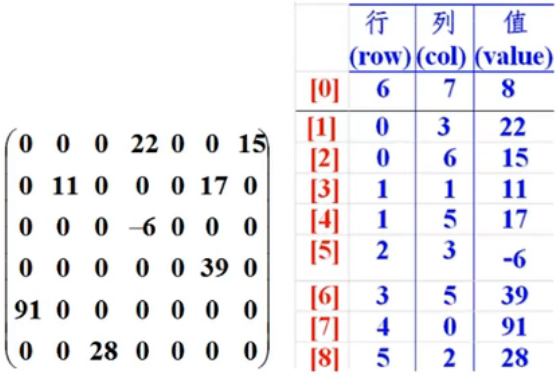### 示例代码：

``````public class ArrayDemo08 {
public static void main(String[] args) {
//1．创建一个二维数组11*110:没有棋子，1:黑棋2:白棋3
int[][] array1 = new int;
array1 = 1;
array1 = 2;
//输出原始的数组
System.out.println("输出原始的数组：");
for (int[] ints : array1) {
for (int anInt : ints) {
System.out.print(anInt + "\t");
}
System.out.println();
}

System.out.println("=====================");

//转换为稀疏数组保存
//获取有效值的个数
int sum = 0;
for (int i = 0; i < 11; i++) {
for (int j = 0; j < 11; j++) {
if (array1[i][j] != 0) {
sum++;
}
}
}
System.out.println("有效值的个数:" + sum);

// 2.创建一个稀疏数组的数组
int[][] array2 = new int[sum + 1];
array2 = 11;
array2 = 11;
array2 = sum;
//遍历二维数组，将非零的值，存放稀疏数组中
int count = 0;
for (int i = 0; i < array1.length; i++) {
for (int j = 0; j < array1[i].length; j++) {
if (array1[i][j] != 0) {
count++;
array2[count] = i;
array2[count] = j;
array2[count] = array1[i][j];
}
}
}
// 输出稀疏数组
System.out.println("稀疏数组数据：");

for (int i = 0; i < array2.length; i++) {
System.out.println(array2[i] +"行"+ "\t"
+ array2[i] +"列"+ "\t"
+ array2[i] +"个"+ "\t");
}

System.out.println("=====================");
System.out.println("还原稀疏数组");

// 1.读取稀疏数组
int[][] array3 = new int[array2][array2];
//2.给其中的元素还原它的值
for (int i = 1; i < array2.length; i++) {
array3[array2[i]][array2[i]] = array2[i];
}

//3.打印
System.out.println("输出还原的数组：");

for (int[] ints : array3) {
for (int anInt : ints) {
System.out.print(anInt + "\t");
}
System.out.println();
}

}
}``````
4

### 评论 (1)

OwO

1.这么多东西，可惜了没人看